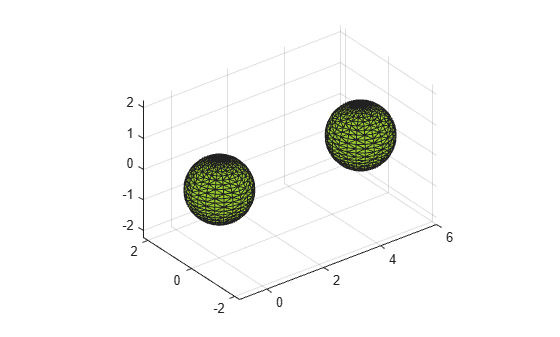surfaceArea

Surface area of 3-D alpha shape

Description

example

A = surfaceArea(shp) returns the total surface area of 3-D alpha shape shp, including the surface area of any interior voids in the alpha shape.

example

A = surfaceArea(shp,RegionID) returns the surface area of a region of the alpha shape. RegionID is the ID for the region and 1RegionIDnumRegions(shp).

Examples

collapse all

Create a set of 3-D points.

[x1, y1, z1] = sphere(24);
x1 = x1(:);
y1 = y1(:);
z1 = z1(:);
x2 = x1+5;
P = [x1 y1 z1; x2 y1 z1];
P = unique(P,'rows');

Create and plot an alpha shape using an alpha radius of 1.5.

shp = alphaShape(P,1.5);
plot(shp)Compute the surface area of the alpha shape.

totalsurfarea = surfaceArea(shp)
totalsurfarea = 24.9361

Compute the surface area of each region separately.

regionsurfareas = surfaceArea(shp, 1:numRegions(shp))
regionsurfareas = 1×2

12.4680   12.4680

Input Arguments

collapse all

3-D alpha shape, specified as an alphaShape object. For more information, see alphaShape

Example: shp = alphaShape(x,y,z) creates a 3-D alphaShape object from the (x,y,z) point coordinates.

ID number for region in alpha shape, specified as a positive integer scalar between 1 and numRegions(shp).

An alpha shape can contain several smaller regions, depending on the point set and parameters. Each of these smaller regions is assigned a unique RegionID, which numbers the regions from the largest area or volume to the smallest. For example, consider a 3-D alpha shape with two regions. The region with the largest volume has a RegionID of 1, and the smaller region has a RegionID of 2.

Example: shp.RegionThreshold = area(shp,numRegions(shp)-2); suppresses the two smallest regions in 2-D alpha shape shp.

Data Types: double